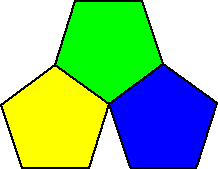Squares? Yep!What happens at each vertex?

90 + 90 + 90 + 90 = 360 degrees again!

So, we need to use regular polygons that add up to 360 degrees.

Will pentagons work?

The interior angle of a pentagon is 108 degrees. . .

108 + 108 + 108 = 324 degrees . . . Nope!Hexagons?

120 + 120 + 120 = 360 degrees Yep!